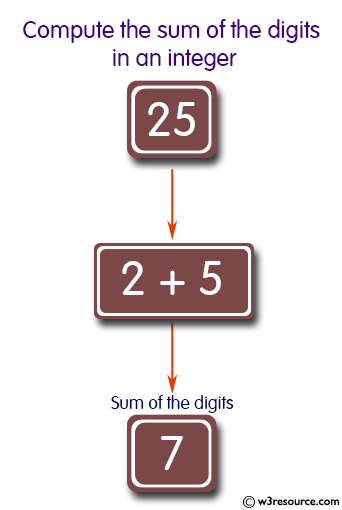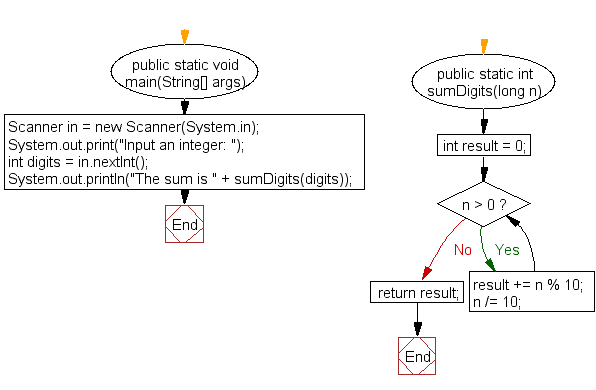﻿ Java exercises: Compute the sum of the digits in an integer - w3resource# Java Method Exercises: Compute the sum of the digits in an integer

## Java Method: Exercise-6 with Solution

Write a Java method to compute the sum of the digits in an integer.

Test Data:
Input an integer: 25

Pictorial Presentation:Sample Solution:

Java Code:

``````import java.util.Scanner;
public class Exercise6 {

public static void main(String[] args)
{
Scanner in = new Scanner(System.in);
System.out.print("Input an integer: ");
int digits = in.nextInt();
System.out.println("The sum is " + sumDigits(digits));
}

public static int sumDigits(long n) {
int result = 0;

while(n > 0) {
result += n % 10;
n /= 10;
}

return result;
}

}
```
```

Sample Output:

```Input an integer: 25
The sum is 7
```

Flowchart:Java Code Editor: